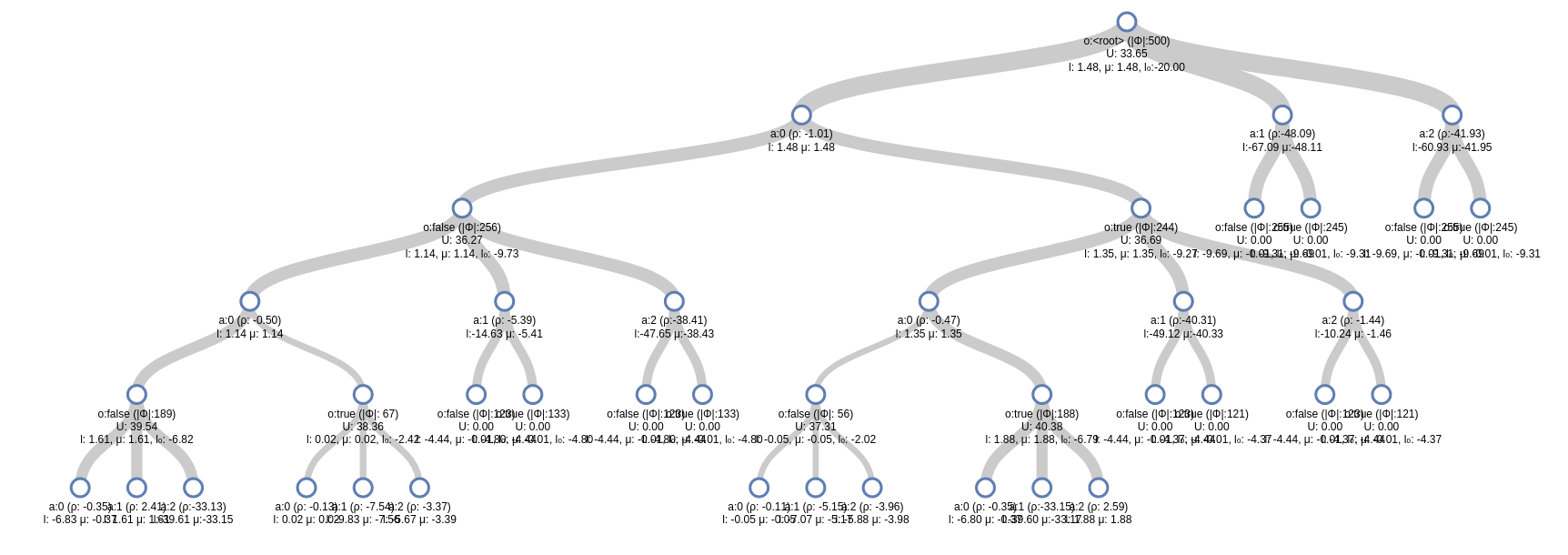6

6

5

5

# ARDESPOT

An implementation of the AR-DESPOT (Anytime Regularized DEterminized Sparse Partially Observable Tree) online POMDP Solver.

Tried to match the pseudocode from this paper: http://bigbird.comp.nus.edu.sg/m2ap/wordpress/wp-content/uploads/2017/08/jair14.pdf as closely as possible. Look there for definitions of all symbols.

Problems use the POMDPs.jl generative interface.

If you are trying to use this package and require more documentation, please file an issue!

## Installation

On Julia v0.7 or later, ARDESPOT is in the JuliaPOMDP registry

``````Pkg.add("POMDPs")
using POMDPs
``````

## Usage

``````using POMDPs, POMDPModels, POMDPSimulators, ARDESPOT

pomdp = TigerPOMDP()

solver = DESPOTSolver(bounds=(-20.0, 0.0))
planner = solve(solver, pomdp)

for (s, a, o) in stepthrough(pomdp, planner, "s,a,o", max_steps=10)
println("State was \$s,")
println("action \$a was taken,")
end
``````

For minimal examples of problem implementations, see this notebook and the POMDPs.jl generative docs.

## Solver Options

Solver options can be found in the `DESPOTSolver` docstring:

``````julia> ?DESPOTSolver
...
epsilon_0       :: Float64
xi              :: Float64
K               :: Int64
D               :: Int64
lambda          :: Float64
T_max           :: Float64
max_trials      :: Int64
bounds          :: Any
default_action  :: Any
rng             :: AbstractRNG
random_source   :: ARDESPOT.DESPOTRandomSource
bounds_warnings :: Bool
``````

Each can be set with a keyword argument in the DESPOTSolver constructor. The definitions of the parameters match as closely as possible to the corresponding definition in the pseudocode of this paper.

### Bounds

Bounds can be specified as a tuple `(lower_bound, upper_bound)`, where the lower and upper bounds are one of

• a number
• a function `f` - in this case `f(pomdp, b::ScenarioBelief)` is called to determine the bound.
• an object `bound` - in this case `ARDESPOT.lbound(bound, pomdp, b::ScenarioBelief)` or `ARDESPOT.ubound(...)` will be called to determine the bound.

To access the state particles in a `ScenairoBelief` `b`, use `particles(b)` (or `collect(particles(b))` to get a vector).

A common lower bound strategy is to use a rollout policy. This is provided by the `DefaultPolicyLB` type, for example

``````DESPOTSolver(bounds=(DefaultPolicyLB(RandomSolver()), 0.0))
``````

will use a random rollout policy to calculate a lower bound.

Using an `IndependentBounds` object adds some robustness. For example

``````DESPOTSolver(bounds=IndependentBounds(DefaultPolicyLB(RandomSolver()), 0.0, check_terminal=true, consistency_fix_thresh=0.1))
``````

will automatically correct some common problems arising from using approximate bounds.

Bounds need not be calculated independently; a single object or function that returns a tuple can be passed to the `bounds` argument. More examples can be found in src/bounds.jl. Please file an issue if more documentation is needed.

## Visualization

D3Trees.jl can be used to visualize the search tree, for example

``````using POMDPs, POMDPModels, POMDPModelTools, D3Trees, ARDESPOT

pomdp = TigerPOMDP()

solver = DESPOTSolver(bounds=(-20.0, 0.0), tree_in_info=true)
planner = solve(solver, pomdp)
b0 = initialstate_distribution(pomdp)

a, info = action_info(planner, b0)
inchrome(D3Tree(info[:tree], init_expand=5))
``````

will create an interactive tree that looks like this:## Relationship to DESPOT.jl

DESPOT.jl was designed to exactly emulate the C++ code released by the original DESPOT developers. This implementation was designed to be as close to the pseudocode from the journal paper as possible for the sake of readability. ARDESPOT has a few more features (for example DESPOT.jl does not implement regularization and pruning), and has more compatibility with a wider range of POMDPs.jl problems because it does not emulate the C++ code.

08/16/2017

8 months ago

99 commits